# JP2005006188A - Crc computation method and crc computing unit - Google Patents

## Info

Publication number
JP2005006188A
JP2005006188A JP2003169574A JP2003169574A JP2005006188A JP 2005006188 A JP2005006188 A JP 2005006188A JP 2003169574 A JP2003169574 A JP 2003169574A JP 2003169574 A JP2003169574 A JP 2003169574A JP 2005006188 A JP2005006188 A JP 2005006188A
Authority
JP
Japan
Prior art keywords
crc
division
polynomial
multiplication
performed
Prior art date
Legal status (The legal status is an assumption and is not a legal conclusion. Google has not performed a legal analysis and makes no representation as to the accuracy of the status listed.)
Abandoned
Application number
JP2003169574A
Other languages
Japanese (ja)
Inventor
Yoshikuni Miyata
Takahiko Nakamura
Rui Sakai
Hideo Yoshida

Original Assignee
Mitsubishi Electric Corp

Priority date (The priority date is an assumption and is not a legal conclusion. Google has not performed a legal analysis and makes no representation as to the accuracy of the date listed.)
Filing date
Publication date
Application filed by Mitsubishi Electric Corp, 三菱電機株式会社 filed Critical Mitsubishi Electric Corp
Priority to JP2003169574A priority Critical patent/JP2005006188A/en
Publication of JP2005006188A publication Critical patent/JP2005006188A/en
Application status is Abandoned legal-status Critical

• 238000007792 addition Methods 0 abstract 3
• 230000001976 improved Effects 0 abstract 1

## Images

•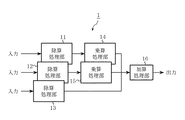•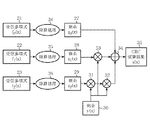•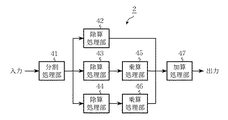•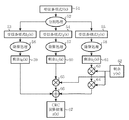••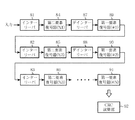•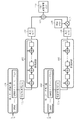••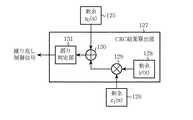•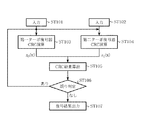•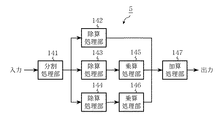•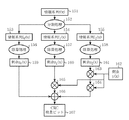••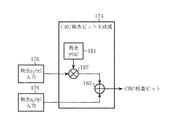•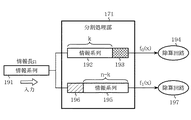•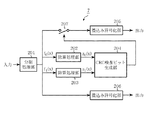•## Abstract

<P>PROBLEM TO BE SOLVED: To provide a CRC computing unit with improved speed of computation by performing multiplication and addition for degree adjustment, after conducting a division operation on each input data in parallel based on a CRC (Cyclic Redundancy Check) generating polynomial for data inputted in parallel. <P>SOLUTION: A configuration of a CRC computing unit 1 for a case when three inputs of divided received polynomials are in parallel comprises division processing units 11, 12 and 13 for conducting division for each received polynomial inputted based on a CRC generating polynomial, multiplication processing units 14 and 15 for conducting multiplication for a remainder as a result of each division to adjust the degree of the received polynomials, and an addition processing unit 16 for conducting addition of remainders performed degree adjustment. <P>COPYRIGHT: (C)2005,JPO&NCIPI

## Description


BACKGROUND OF THE INVENTION
The present invention relates to a CRC calculation method and a CRC calculation device related to CRC (Cyclic Redundancy Check) calculation for detecting an error in digital information.

[Prior art]
The CRC code is generated by adding a remainder of the information sequence i (x) by the generating polynomial g (x) to the information sequence. To add an n-bit CRC check bit, an n-th generation polynomial g (x) is prepared, a remainder v (x) of g (x) of i (x) is obtained, and v (x) is used as a check bit. Append.

When performing error detection, i (x) · x n The remainder z (x) is obtained from the generating polynomial g (x) of the receiving polynomial f (x) corresponding to + v (x). If there is no error in the reception polynomial r (x), r (x) = i (x) · x n + V (x) is divisible by the generator polynomial g (x), and the remainder z (x) is 0. If there is an error, it is not divisible and 0, so it is possible to detect an error (for example, non-patent Reference 1).

Since the CRC operation for generating and checking the CRC check bits is division based on a generator polynomial, it can be configured using a shift register. Further, it is possible to speed up the calculation by reading and calculating the data polynomial in a plurality of bits in parallel.

A conventional speeding-up method by parallelizing CRC operations is described in Patent Document 1. The basic calculation method realizes parallelization by multiplying data input in parallel by a power to adjust the degree of the data polynomial in advance, dividing the result, and adding the remainder. For example, a polynomial is expanded by equation (1), and f 1 (X) and f 3 X in (x) 32 , The remainder from each generator polynomial g (x) is obtained, and each is added. A feature is that division is performed after multiplication in advance.
[Expression 1]

[Non-Patent Document 1]
"Code Theory" IEICE (p.118)
[Patent Document 1]
JP-A-8-330976

[Problems to be solved by the invention]
In the conventional CRC calculation, since the reception polynomial is input to the division circuit in order from the higher order, the number of steps for the code length is required for processing, and there is a problem that the delay time is large. Further, when division is performed on a plurality of reception polynomial blocks, it is necessary to perform division from a higher order of the reception polynomial, and therefore, a lower order reception polynomial block cannot be started unless the higher order calculation is completed. Therefore, the processing delay for performing the CRC operation on the received signal is required for the division for all the codes.

Therefore, a method has been proposed in which CRC operations are parallelized to increase the operation speed, but this is a method of multiplying a received polynomial block in advance and then performing a division process. Since it is difficult to multiply the data due to restrictions such as the above, it is difficult to cope with a case where the size of one block to be divided is limited and the size of one block changes. Conversely, when one block is made small, the number of parallelization increases when the code length is long, and the circuit scale becomes large. Further, when a polynomial for performing CRC calculation is input in parallel bit by bit, it is necessary to hold information up to the data size unit to be read.

In addition, when a concatenated code of a turbo code and a CRC code is used and there are a plurality of reception polynomials, it is necessary to rearrange the data polynomial at the end of the turbo decoding operation. If decoding for all data is not completed, the CRC operation is performed. Cannot start. Even if turbo decoding with a reversed decoding order is applied, if there are multiple reception polynomials, division for low-order reception polynomials must wait for the completion of high-order computation, and only the division for all codes is processed. There was a problem of taking time.

The present invention has been made to solve the above-described problems. After performing a division operation based on a CRC generator polynomial on data input in parallel to each input data, multiplication and addition of degree adjustment are performed. The purpose of this is to speed up the computation.

[Means for Solving the Problems]
In the CRC calculation method according to the present invention, the reception polynomial is divided into a plurality of blocks and input in parallel, and a division operation based on the CRC generation polynomial is performed in parallel on each reception polynomial, and the result of the division operation is received. After performing multiplication for adjusting the order of the polynomial block, each of the multiplication results is added to obtain a CRC result for the reception polynomial.

In the CRC calculation method according to the present invention, the reception polynomial is divided into a plurality of reception polynomial blocks, division operations based on the CRC generation polynomial are performed in parallel, and the order of the reception polynomial block is adjusted to the result of the division operation. After multiplication, each of the multiplication results is added to obtain a CRC result for all reception polynomials.

In the CRC calculation apparatus according to the present invention, a plurality of division circuits based on a CRC generation polynomial, a multiplier that performs multiplication for adjusting the order of the remainder, and addition are performed in accordance with received data input in parallel And an adder.

In the CRC calculation method according to the present invention, an information sequence is divided into a plurality of blocks and input in parallel, and a division operation based on a CRC generator polynomial is performed on each information sequence block in parallel, and the result of the division operation is obtained. After performing multiplication for adjusting the order of the information sequence block, each of the multiplication results is added to generate a CRC check bit.

In the CRC calculation method according to the present invention, an information sequence is divided into a plurality of blocks, division operations based on a CRC generator polynomial are performed in parallel, and multiplication and addition are performed to match the degree of each block to the remainder of the division operation. Thus, a CRC check bit is obtained.

A CRC calculation apparatus according to the present invention includes a division processing unit that divides an information sequence into a plurality of blocks, a plurality of division units based on a CRC generator polynomial, a multiplication unit that performs multiplication for adjusting a degree of a remainder, and the multiplication Adding means for adding multiplication results by the means.

DETAILED DESCRIPTION OF THE INVENTION
An embodiment of the present invention will be described below.
Embodiment 1 FIG.
Embodiment 1 according to the present invention will be described with reference to FIG. 1 and FIG. FIG. 1 is a diagram showing a circuit configuration of the CRC computing device 1 according to the first embodiment, and FIG. 2 is a block diagram showing an operation of the CRC computing device 1.

The parallelization of CRC calculation by the CRC calculation method of the present invention is based on the following polynomial division method. The value to be obtained by the CRC calculation is a remainder z (x) by the generator polynomial g (x) with respect to the polynomial f (x) and can be expressed by Expression (2).
[Expression 2]
Here, the polynomial f (x) is expanded as shown in Equation (3).
[Equation 3]

Expression (3) can also be regarded as dividing the polynomial f (x) into m, and the size of one divided block is N. Furthermore, the polynomial block f i (X) and power x N When the remainder by the generator polynomial g (x) is expressed by Equation (4) and Equation (5)
[Expression 4]
[Equation 5]
The remainder of the generator polynomial g (x) of Expression (3) can be expressed as Expression (6) using Expression (4) and Expression (5), and the remainder z (x) of the polynomial f (x) is (7)
[Formula 6]
[Expression 7]

From equation (7), each remainder z of the divided polynomial block i It can be seen that the remainder z (x) of the generator polynomial g (x) of the total polynomial f (x) is obtained by multiplying (x) by y (x) for adjusting the order and adding them.

Next, the above will be applied to CRC calculation, and a specific example will be described. The first embodiment is intended to perform division on received data input in parallel in parallel. The CRC calculation device 1 shown in FIG. 1 is a configuration example in which three reception polynomial inputs are arranged in parallel, and division processing units 11, 12, 13 that perform division based on the CRC generation polynomial for each input reception polynomial. The multiplication processing units 14 and 15 that perform multiplication for adjusting the order of the reception polynomial are added to the remainders that are the respective division results, and the addition processing unit 16 that performs addition of the remainders after the order adjustment.

The CRC calculation apparatus 1 according to the first embodiment performs a division process on each of a plurality of input reception polynomial blocks in parallel, and multiplies and adds the results to obtain a CRC calculation result. It is. The division circuit constituting each division processing unit 11 to 13 is constituted by a shift register, and the multiplier constituting the multiplication processing units 14 and 15 is GF (2) modulo a generator polynomial using an exclusive OR circuit. ) The above multiplication circuit is provided.

Next, the operation of the CRC calculation device 1 will be described. FIG. 2 is a block diagram showing the operation of the CRC calculation device 1, and shows a case where the code is divided into three as an example. This operation is generally performed in the following four steps.

First step.
Divided reception polynomial f 0 (X) 21, f 1 (X) 22, f 2 (X) 23 is input to the CRC calculation device 1 in parallel. Each receiving polynomial f 0 (X) 21, f 1 (X) 22, f 2 The relationship between (x) 23 and the total reception polynomial is expressed by equation (3).

Second stroke.
Each receiving polynomial f 0 (X) 21, f 1 (X) 22, f 2 (X) 23 is input to the division circuit in order from each higher order, whereby division processing 24, 25, and 26 are performed in parallel. When all the data of each reception polynomial block has been input, the state of the shift register that constitutes the circuit of each division process 24-25 indicates the remainder by the generator polynomial g (x) of each block, and the result is Remainder z shown in equation (4) i (X) [z 0 (X) 27, z 1 (X) 28, z 2 (X) 29].

Third process.
Remainder z obtained in the second process i In order to match the degree to (x), multiplication processes 31, 32, and 33 with y (x) 30 are performed according to equation (7). Since y (x) 30 can be obtained if the size N of one block of the reception polynomial is determined, it is calculated in advance.

Originally f is a shift register i (X) x i ・ N (I + 1) N clocks are required to obtain the remainder using the generator polynomial g (x), but the remainder z has only N computations, so the remainder z i In (x), the division has not progressed to the requested order. So x N Y (x) 30 shown in the equation (5), which is a remainder of the generator polynomial g (x), is represented on the GF (2) modulo the generator polynomial g (x). i By multiplying (x), it is possible to obtain the same result as the result of the remaining calculation by the shift register. If the operation by the shift register is not i · N times, y (x) 30 is the remainder z i All orders can be adjusted by multiplying (x) i times.

Fourth process.
Finally, if all the multiplication results performed in the third step are subjected to addition processing 34 as shown in equation (7), z (x) which is the CRC calculation result 35 shown in equation (2) is obtained. Can do.

As described above, according to the first embodiment, the division process is divided and performed in parallel, so that the low-order reception polynomial block does not need to wait for the completion of the division of the high-order block, and the process is performed at high speed. If the reception polynomial is output in order from the higher order in each decoding, it is possible to perform CRC calculation simultaneously with decoding by inputting each result to the sequential division circuit.

In addition, since each reception polynomial block is first divided and then multiplied, multiplication is performed by a polynomial less than the order of the generator polynomial, and the size of each reception polynomial block is large or changes. In addition, there is an effect that the circuit scale can be reduced without requiring a large memory or circuit.

Embodiment 2. FIG.
A second embodiment according to the present invention will be described with reference to FIGS. 3 is a diagram showing a circuit configuration of the CRC calculation device 2 according to the second embodiment, and FIG. 4 is a block diagram showing an operation of the CRC calculation device 2.

The second embodiment is different from the first embodiment in that the CRC calculation is parallelized by dividing the reception polynomial. FIG. 3 shows the CRC calculation device 2 according to the second embodiment, which is a configuration example when the reception polynomial is divided into three. Division processing unit 41 that divides the reception polynomial into a plurality of blocks according to Equation (3), division processing units 42, 43, and 44 that perform division based on the CRC generation polynomial, and multiplication of degree adjustment for the remainder that is the division result Multiplication processing units 45 and 46 for performing the above and an addition processing unit 47 for performing the addition thereof. The second embodiment is a CRC arithmetic unit that obtains a CRC result by dividing an input reception polynomial into a plurality of blocks, performing division processing on each block, and performing multiplication and addition on the result.

When dividing the reception polynomial, the address read from the memory is controlled according to the division, the division circuit of each division processing unit 42 to 44 is configured by a shift register, and the multiplier uses an exclusive OR circuit. A multiplication circuit on GF (2) modulo the generator polynomial is provided.

Next, the operation of the CRC calculation device 2 will be described. FIG. 4 is a block diagram showing the operation of the CRC calculation device 2, and shows a case where the code is divided into three as an example. This operation is generally performed in the following five steps.

First step.
A receiving polynomial f (x) 51 is input to an apparatus having a plurality of division circuits, and division processing 52 is performed according to the equation (3) so that the number is equal to the number of division circuits.

Second stroke.
Divided reception polynomial f 0 (X) 53, f 1 (X) 54, f 2 (X) 55 is input in parallel to the respective divider circuits.

Third process.
Each receiving polynomial f 0 (X) 53, f 1 (X) 54, f 2 By inputting (x) 55 to the division circuit in order from each higher order, each division processing 56, 57, 58 is performed in parallel. When all the data of each reception polynomial block has been input, the state of the shift register that constitutes the circuit of each division process 56 to 58 indicates the remainder by the generator polynomial g (x) of each block, and the result is Remainder z shown in equation (4) i (X) [z 0 (X) 59, z 1 (X) 60, z 2 (X) 61].

Fourth process.
Remainder z obtained in the third process i In order to match the order to (x), multiplication processes 63, 64, and 65 with y (x) 62 are performed according to equation (7). Since y (x) 62 can be obtained if the size N of one block of the reception polynomial is determined, it is calculated in advance.

Originally f is a shift register i (X) x i ・ N (I + 1) N clocks are required to obtain the remainder using the generator polynomial g (x), but since the operation in the third step only performs N operations, the remainder z i In (x), the division has not progressed to the requested order. So x N Y (x) 62 shown in the equation (5), which is a remainder of the generator polynomial g (x), is represented on the GF (2) modulo the generator polynomial g (x). i By multiplying (x), it is possible to obtain the same result as the result of the remaining calculation by the shift register. If the operation by the shift register is not i · N times, y (x) 62 is the remainder z i All orders can be adjusted by multiplying (x) i times.

5th process.
Finally, if all the multiplication results performed in the fourth step are subjected to addition processing 66 as shown in equation (7), z (x) which is a CRC calculation result 67 shown in equation (2) is obtained. Can do.

As described above, according to the second embodiment, the received reception polynomial is divided and divided, and the CRC calculation related to the divided low-order reception polynomial block is waited for the completion of the high-order calculation. Since it is not necessary, there is an effect that processing can be performed at high speed.

Embodiment 3 FIG.
Embodiment 3 according to the present invention will be described with reference to FIGS. 5 is a diagram illustrating a circuit configuration of the CRC calculation device 3 according to the third embodiment, FIG. 6 is a diagram illustrating a configuration of a turbo decoding unit used in the CRC calculation device 3, and FIG. FIG. 6 is a diagram illustrating an operation of the arithmetic device 3.

The CRC calculation device 3 according to the third embodiment aims at speeding up calculation when turbo decoding is performed in parallel on a plurality of received sequences and a residual error is detected by CRC thereafter. FIG. 5 is a configuration diagram of the CRC calculation device 3 and shows an example in which two are parallelized. Turbo decoding units 71 and 72 that perform turbo decoding and a division processing unit 73 that performs division based on a CRC generation polynomial. 74, a multiplication processing unit 75 for multiplying each division result by degree adjustment, and an addition processing unit 76 for adding them.

The division circuit constituting the division processing units 73 and 74 is formed of a shift register, and the multiplier includes a multiplication circuit on GF (2) modulo a generator polynomial using an exclusive OR circuit.

In addition, if a turbo decoding method in which the decoding calculation order is changed is used for turbo decoding, calculation processing can be performed at higher speed. The turbo decoding whose order has been changed is the operation of the first element decoder for calculating the likelihood of the non-interleaved received sequence, first the operation of the second element decoder for calculating the likelihood of the interleaved received sequence. A turbo decoding method which is performed later, and performs path metric calculation for the number of information bits n in each element decoder, first performing backward path metric calculation from time point n to 1, and later performing forward path metric calculation from time point 1 to n It is.

In this turbo decoding method, as shown in FIG. 6, first, input data is interleaved by an interleaver 81, then decoded by a second element decoder 84, and then deinterleaved by a deinterleaver 87 to be first element decoded. Decoder 89 decodes the data. By performing this processing up to the first element decoder 91, in order to calculate the path metric from the time point 1 to n in the state where the final iteration of the turbo decoding is not interleaved, the decoding result is It is output from higher order. By doing so, the decoding results are output in descending order from the higher order of the reception polynomial, and the results can be input to the CRC calculation unit 92 at any time.

In FIG. 6, the symbol “%” of the second element decoders 84 to 86 represents the decoding process of the interleaved sequence, and the symbol “#” of the first element decoders 89 to 91 represents the decoding of the sequence that has not been interleaved. The number following the symbols “%” and “#” represents the number of turbo decoding iterations. For example, # 2 represents the decoding process of the sequence that is the second time the turbo decoding iteration is repeated and is not interleaved.

Next, the operation of the CRC calculation device 3 will be described. FIG. 7 is a block diagram showing the operation of the CRC calculation device 3, and shows a case where it is divided into two as an example. This operation is generally performed in the following four steps.

First step.
The received sequence is input to the first turbo decoder 101 and the second turbo decoder 102 to perform decoding in parallel. When the turbo decoding method with the order changed is used, the decoding result is the reception polynomial f. 0 (X) 103, f 1 (X) The decoding results are output bit by bit from the higher order of 104 in descending order.

Second stroke.
Reception polynomial f that is output bit by bit from the higher order in the first step 0 (X) 103, f 1 (X) 104 is input to the dividing circuit 105 and the dividing circuit 106 as needed, so that each division is processed in parallel. Further, since the turbo decoding result is immediately input to the division circuit, the final round of turbo decoding and the division can be parallelized. When turbo decoding is completed and all the reception polynomial blocks have been input, the state of the shift register that constitutes each of the division circuits 105 and 106 indicates a remainder from the generation polynomial g (x) of each block. Next, the remainder z shown in equation (4) i (X) [z 0 (X) 107, z 1 (X) 108] is read out.

Third process.
Remainder z obtained in the second process i In order to match the degree to (x), multiplication processing 110 with y (x) 109 is performed according to the equation (7). Since y (x) 109 can be obtained if the size N of one block of the reception polynomial is determined, it is calculated in advance.

Originally f is a shift register i (X) x i ・ N (I + 1) N clocks are required to obtain the remainder using the generator polynomial g (x), but the remainder z has only N computations, so the remainder z i In (x), the division has not progressed to the requested order. So x N Y (x) 109 shown in the equation (5), which is a remainder by the generator polynomial g (x) of, modulo the generator polynomial g (x) on GF (2), and the remainder z i By multiplying (x), it is possible to obtain the same result as the result of the remaining calculation by the shift register. If the operation by the shift register is not i · N times, y (x) 109 is the remainder z i All orders can be adjusted by multiplying (x) i times.

Fourth process.
Finally, if all the results of multiplication performed in the third step are subjected to addition processing 111 as shown in equation (7), z (x) which is the CRC calculation result 112 shown in equation (2) is obtained. Can do.

As described above, according to the third embodiment, by combining a parallel CRC calculation and a turbo decoding method in which the decoding order is switched, a multi-input reception polynomial is interleaved in the final round of turbo decoding. When there is no decoding, the CRC calculation can be performed at the same time, the delay time by the CRC calculation can be greatly reduced, and after the turbo decoding is finished, the CRC result can be obtained only by the delay time by the multiplication and addition of the order adjustment, There is an effect that processing can be performed at high speed.

Embodiment 4 FIG.
Embodiment 4 according to the present invention will be described with reference to FIGS. 8 is a block diagram showing the configuration of the CRC calculation device 4 according to Embodiment 4, FIG. 9 is a diagram showing the configuration of the CRC result calculation unit shown in FIG. 8, and FIG. 10 is this CRC calculation device. 4 is a flowchart showing a flow of operation of FIG.

As shown in FIGS. 8 and 9, the CRC calculation device 4 according to the fourth embodiment is a case in which CRC calculation is performed while turbo decoding is performed in parallel on a plurality of received sequences to control the number of turbo decoding iterations. The CRC calculation device 4 is shown as an example in which the CRC calculation device 4 is parallelized, and includes a first turbo decoder 121 and a second turbo decoder 122 that perform turbo decoding. Further, the remainder z is obtained from the output of the first turbo decoder 121 based on the CRC generator polynomial. 0 (X) Divide circuit 123 for calculating 125 and the remainder z from the output of second turbo decoder 122 1 (X) A division circuit 124 for calculating 126 is provided, and the remainder z 1 (X) 126 is subjected to degree adjustment multiplication processing 129, and the processing result and the remainder z 0 (X) A CRC result calculation unit 127 is provided that calculates the CRC result by adding 130 and performs error determination based on the CRC result. The CRC result calculation unit 127 includes an error determination unit 131. The error determination unit 131 determines the presence / absence of an error from the CRC result, and outputs a control signal for post-determination turbo decoding repetition calculation.

If turbo decoding with a reversed order is applied to turbo decoding, the processing can be further speeded up. The turbo decoding whose order has been changed is that the operation of the second element decoder 84 for calculating the likelihood of the interleaved received sequence is performed first, and the likelihood of the received sequence not interleaved is calculated by the first element decoder 89. The calculation is performed later, the path metric calculation for the number of information bits n in the second element decoder 84 and the first element decoder 89 is performed, the backward path metric calculation from time n to 1 is performed first, and from time 1 to n This is a turbo decoding method in which a forward path metric calculation is performed later.

The CRC result calculation unit 127 determines the presence or absence of an error from the CRC calculation result and repeatedly outputs a control signal. The repetitive control signal is reflected in the turbo decoding iterative calculation. If there is no error, the decoding is stopped, and if it is, the repetitive decoding is continued. The division circuit 123 and the division circuit 124 are configured by shift registers, and the CRC result calculation unit 127 includes a multiplication circuit on GF (2) modulo a generator polynomial using an exclusive OR circuit as a multiplier. ing.

Next, the operation of the CRC calculation device 4 will be described. FIG. 10 is a flowchart showing the flow of the operation of the CRC arithmetic unit 4, and shows a case where it is divided into two as an example. This operation is generally performed in the following four steps.

First step.
The received sequence is input to first turbo decoder 121 and second turbo decoder 122, respectively (step ST101 and step ST102).

Second stroke.
Next, decoding by the first turbo decoder 121 and division that is part of the CRC calculation are performed (step ST103), and decoding by the second turbo decoder 122 and division that is part of the CRC calculation are performed (step ST104). Since decoding and division are performed simultaneously, they are shown together in the flowchart of FIG. Step ST103 and step ST104 are performed simultaneously.

The first turbo decoder 121 and the second turbo decoder 122 first perform decoding in an interleaved state, and then perform decoding in a non-interleaved state. Since the decoding results in the non-interleaved state are output in descending order from the higher order of the reception polynomial, the results are input to the dividing circuit 123 and the dividing circuit 124 as needed. By inputting the decoding result to the division circuit as needed, division and turbo decoding can be performed in parallel. When decoding is completed, division is also completed.

Third process.
The state of the shift register that constitutes the division circuits 123 and 124 indicates the remainder by the generator polynomial g (x) of each block, and as a result, z shown in Expression (4) i (X) [z 0 (X) 125, z 1 (X) 126] is read out and input to the CRC result calculation unit 127. In the CRC result calculation unit 127, each division result z obtained in the second step i In order to match the degree of the remainder to (x), the CRC result is calculated by multiplying and adding y (x) 128 shown in Expression (5) (step ST105), and the error determination unit 131 determines whether there is an error. Is determined (step ST106), and a control signal for iterative decoding of turbo decoding is output. The processing time required during this time is only several clocks for judging multiplication, addition and error. Since y (x) 128 can be obtained once the size N of one reception polynomial block is determined, it is calculated in advance.

Originally f is a shift register i (X) x i ・ N (I + 1) N clocks are required to obtain the remainder using the generator polynomial g (x), but the remainder z has only N computations, so the remainder z i In (x), the division has not progressed to the requested order. So x N Y (x) 128 shown in the equation (5), which is a remainder of the generator polynomial g (x), is represented on the GF (2) modulo the generator polynomial g (x). i By multiplying (x), it is possible to obtain the same result as the result of the remaining calculation by the shift register. If the operation by the shift register is not i · N times, y (x) 128 is the remainder z i All orders can be adjusted by multiplying (x) i times.

Fourth process.
If there is an error in step ST106, the turbo decoding is repeated from step ST103 and step ST104. On the other hand, if there is no error, the decoding result is output (step ST107). Note that the maximum number of repetitions may be set, and the repetition calculation may be terminated at a predetermined number of times.

As described above, according to the fourth embodiment, when the number of iterations of turbo decoding is controlled from the CRC calculation result, the CRC calculation is performed by combining the CRC calculation parallelization and the turbo decoding method in which the decoding order is switched. The delay time is limited only to multiplication, addition and error determination for adjusting the order of the reception polynomial block, and it is possible to perform the number of repetitions control with a delay time shorter than the conventional one, and there is an effect that the control can be performed at high speed.

Embodiment 5 FIG.
Embodiment 5 according to the present invention will be described with reference to FIGS. FIG. 11 is a diagram showing a circuit configuration of the CRC calculation device 5 according to the fifth embodiment, and FIG. 12 is a block diagram showing an operation of the CRC calculation device 5.

The CRC computing device 5 according to the fifth embodiment is intended to obtain CRC check bits at high speed. FIG. 11 shows the configuration of the CRC calculation device 5 according to the fifth embodiment, which is an example in the case of division into three, a division processing unit 141 that divides an information sequence into a plurality of blocks according to equation (3), and a CRC generator polynomial. Division processing units 142, 143, 144 that perform division by, multiplication processing units 145, 146 that perform multiplication for adjusting the degree of the remainder in accordance with Expression (7), and an addition processing unit 147 that performs addition thereof. It is a CRC arithmetic unit that generates a CRC check bit by inputting and dividing an information sequence, processing division in parallel for each information sequence, and performing multiplication and addition.

Next, the operation of the CRC calculation device 5 will be described. FIG. 12 is a block diagram showing the CRC calculation device 5, and shows a case where it is divided into three as an example. This operation is generally performed in the following five steps.

First step.
The information series f (x) 151 is input to a device having a plurality of division circuits, and the division processing 152 is performed according to the equation (3) to the same number as the number of division circuits.

Second stroke.
Divided information sequence block f 0 (X) 153, f 1 (X) 154, f 2 (X) 155 is input in order from the higher order of each information sequence block.

Third process.
Each information series block f 0 (X) 153, f 1 (X) 154, f 2 (X) Each division process 156, 157, 158 is performed in parallel by inputting 155 to the division circuit in order from each higher order. At the time when all the data of each information series block has been input, the state of the shift register constituting the circuit of each of the division processes 156 to 158 indicates the remainder by the generator polynomial g (x) of each block, and the result is Remainder z shown in equation (4) i (X) [z 0 (X) 159, z 1 (X) 160, z 2 (X) 161].

Fourth process.
Remainder z obtained in the third process i In order to match the order to (x), multiplication processing 163, 164, and 165 with y (x) 162 is performed according to Expression (7). Since y (x) 162 can be obtained if the size N of one block of the information sequence block is determined, it is calculated in advance.

Originally f is a shift register i (X) x i ・ N (I + 1) N clocks are required to obtain the remainder using the generator polynomial g (x), but since the operation in the third step only performs N operations, the remainder z i In (x), the division has not progressed to the requested order. So x N Y (x) 162 shown in the equation (5), which is a remainder of the generator polynomial g (x), is represented on the GF (2) modulo the generator polynomial g (x). i By multiplying (x), it is possible to obtain the same result as the result of the remaining calculation by the shift register. If the operation by the shift register is not i · N times, y (x) 162 is the remainder z i All orders can be adjusted by multiplying (x) i times.

5th process.
Finally, if all the multiplication results performed in the fourth step are subjected to addition processing 166 as shown in equation (7), z (x) which is a CRC check bit 167 can be obtained.

As described above, according to the fifth embodiment, the processing for adding the CRC check bit is divided and performed in parallel, so that the operation can be processed at high speed.

Embodiment 6 FIG.
Embodiment 6 according to the present invention will be described with reference to FIGS. 13 is a diagram illustrating a circuit configuration of the CRC calculation device 6 according to the sixth embodiment, FIG. 14 is a diagram illustrating a configuration of the CRC check bit generation unit in FIG. 13, and FIG. 15 is a diagram illustrating the CRC calculation device. 6 is a configuration diagram showing a part of FIG.

In the sixth embodiment, it is assumed that CRC check bits are generated from an information sequence, code division is performed on the information sequence to which the CRC check bit is added, and each divided sequence is separately turbo-encoded. is doing. The CRC computing device 6 of the sixth embodiment aims to speed up the CRC check bit generation and encoding processing in the above case. FIG. 13 shows a configuration diagram of the sixth embodiment. A division processing unit 171 that divides an information sequence according to a code division rule, a division processing unit 172 that divides each divided code, a division processing unit 173, A CRC check bit generation unit 174 that performs multiplication and addition on the division result to generate a CRC check bit, a turbo encoding unit 175 that performs turbo encoding, and a turbo encoding unit 176 are provided. The CRC arithmetic unit 6 is a CRC arithmetic unit that divides an information sequence according to a code division rule, performs division in parallel, and calculates and adds a CRC check bit from the remainder. The division circuits of the division processing units 172 and 173 are constituted by shift registers, and the multiplier includes a multiplication circuit on GF (2) modulo a generator polynomial using an exclusive OR circuit.

Next, the operation of the CRC calculation device 6 will be described. Here, the case where it is divided into two is shown as an example. This operation is generally performed in the following four steps.

First step.
First, as shown in FIG. 13, an information series is input to the division processing unit 171 and division processing is performed according to the code division rule. Originally, since code division is performed after the CRC check bit is added, it is necessary to divide the code including the CRC check bit length as shown in FIG. At this time, the adjustment bit 196 in the code division is added, and the CRC check bit 193 is left empty.

When the information sequence 191 having the information length n is input to the division processing unit 171 illustrated in FIG. 15, the information sequence block f input to the division circuit 194 of the division processing unit 172 in consideration of the CRC check bit 193 length. 0 An information sequence 192 to be (x) and an adjustment bit 196 are added, and an information sequence block f input to the division circuit 197 of the division processing unit 173 1 It is divided into information series 195 that becomes (x).

Second stroke.
Each information sequence block f divided in the first step 0 (X), f 1 (X) is input to the division processing unit 172 and the division processing unit 173, respectively, and each division is processed in parallel, and the remainder z shown in Expression (4) is obtained. i (X) [z 0 (X), z 1 (X)] is obtained. In this case, f 0 The division of (x) is completed earlier by the CRC check bit.

Third process.
Remainder z obtained in the second process i (X) is input to the CRC check bit generation unit 174 shown in FIG. 14, and the CRC check bit 193 is obtained. The CRC check bit generation unit 174 first performs a multiplication process 182 with y (x) 181 in accordance with Expression (7) in order to match the degree of the remainder. Since y (x) 181 can be obtained if the size N of one block of the information sequence block is determined, it is calculated in advance.

Originally f is a shift register i (X) x i ・ N (I + 1) N clocks are required to obtain the remainder using the generator polynomial g (x), but the remainder z has only N computations, so the remainder z i In (x), the division has not progressed to the requested order. So x N Y (x) 181 shown in the equation (5), which is a remainder by the generator polynomial g (x) of, and a remainder z on GF (2) modulo the generator polynomial g (x) i By multiplying (x), it is possible to obtain the same result as the result of the remaining calculation by the shift register. If the operation by the shift register is not i · N times, y (x) 181 is the remainder z i All orders can be adjusted by multiplying (x) i times.

Fourth process.
Finally, information sequence block f with CRC check bit 193 added in the third step f 0 (X) is encoded by a turbo encoding unit 175, and an information sequence block f to which an adjustment bit 196 is added 1 (X) is encoded by the turbo encoding unit 176 and output. The SW 177 determines the information sequence block f based on the result of the CRC check bit generation unit 174. 0 Control the encoding of (x).

As described above, according to the sixth embodiment, the CRC check bit generation process can be speeded up by performing the CRC calculation in parallel, which is originally necessary for parallelizing the CRC calculation. By performing the information sequence division processing by code division processing, there is no division processing for CRC parallelization, and there is an effect that computation can be performed at high speed.

Embodiment 7 FIG.
A seventh embodiment according to the present invention will be described with reference to FIGS. 16 is a diagram showing a circuit configuration of the CRC calculation device 7 according to the seventh embodiment, and FIG. 17 is a configuration diagram showing a part of the CRC calculation device 7.

In the seventh embodiment, a CRC check bit is generated from an information sequence, code division is performed on the information sequence to which the CRC check bit is added, and convolutional coding is separately performed on each divided sequence. Assumes. The CRC computing device 7 of the seventh embodiment aims to speed up the CRC check bit generation and encoding processing in the above case. FIG. 16 shows a configuration diagram of Embodiment 7, in which a division processing unit 201 that divides an information sequence according to a code division rule, a division processing unit 202 that divides each divided code, a division processing unit 203, A CRC check bit generation unit 204 that performs multiplication and addition on the division result to generate a CRC check bit, a convolutional coding unit 205 that performs convolutional coding, and a convolutional coding unit 206 are provided. The CRC arithmetic unit is a CRC arithmetic unit that divides an information sequence according to a code division rule, performs division in parallel, and calculates and adds a CRC check bit from the remainder.

Note that the division circuit of each division processing unit 202, 203 is configured by a shift register, and the multiplier includes a multiplication circuit on FG (2) modulo a generator polynomial using an exclusive OR circuit.

Next, the operation of the CRC calculation device 7 will be described. Here, the case where it is divided into two is shown as an example. This operation is generally performed in the following four steps.

First step.
First, as shown in FIG. 16, an information series is input to the division processing unit 201, and division processing is performed according to the code division rule. Originally, since code division is performed after the CRC check bit is added, it is necessary to divide the code including the CRC check bit length as shown in FIG. At this time, the adjustment bit 196 in the code division is added, and the CRC check bit 193 is left empty.

When the information sequence 211 having the information length n is input to the division processing unit 201 illustrated in FIG. 17, the information sequence block f input to the division circuit 214 of the division processing unit 202 in consideration of the CRC check bit 213 length. 0 An information sequence 212 f to which (x) and an adjustment bit 217 are added and input to the division circuit 218 of the division processing unit 203 1 It is divided into information series 216 that becomes (x).

Second stroke.
Each information series block f shown by Formula (3) divided | segmented at the 1st process 0 (X), f 1 (X) is input to the division processing unit 202 and the division processing unit 203, respectively, and each division is processed in parallel, and the remainder z shown in Expression (4) is obtained. i (X) [z 0 (X), z 1 (X)] is obtained. In this case, f 0 The division of (x) is completed earlier by the CRC check bit.

The bits input to the division circuits 214 and 218 are simultaneously input to the convolutional encoder 215 and the convolutional encoder 219, respectively, and are subjected to convolutional encoding in parallel with the division. Where f 0 The convolutional encoding of the CRC check bit part in (x) is performed by inputting the CRC check bit after finishing the processing of the next third step.

Third process.
Remainder z obtained in the second process i The CRC check bit 213 is obtained from (x), which is the same as that described with reference to FIG. 14 in the sixth embodiment.

Originally f is a shift register i (X) x i ・ N (I + 1) N clocks are required to obtain the remainder using the generator polynomial g (x), but the remainder z has only N computations, so the remainder z i In (x), the division has not progressed to the requested order. So x N Y (x) shown in Expression (5), which is a remainder from the generator polynomial g (x), is represented on GF (2) modulo the generator polynomial g (x), and the remainder z i By multiplying (x), it is possible to obtain the same result as the result of the remaining calculation by the shift register. If the operation by the shift register is not i · N times, y (x) is the remainder z i All orders can be adjusted by multiplying (x) i times. In order to obtain the remainder for all information series f (x), if all the multiplication results performed in the third step are added according to equation (7), z (x) which is a CRC check bit can be obtained.

Fourth process.
Information sequence block f with CRC check bit 213 added at the end 0 (X) is encoded by the convolutional encoder 215 of the convolutional encoding unit 205, and the information sequence block f to which the adjustment bit 217 is added 1 (X) is encoded by the convolutional encoder 219 of the convolutional encoding unit 206 and output. The SW 207 calculates the polynomial f based on the result of the CRC check bit generation unit 204. 0 Control the encoding of (x).

As described above, according to the seventh embodiment, by performing CRC calculation and convolutional coding in the case of code division in parallel, the delay time by CRC calculation is reduced, and CRC calculation parallelization is performed. By performing the division processing for the code division processing, there is no division processing for CRC calculation parallelization, and the coding processing can be performed at high speed.

【The invention's effect】
As described above, according to the present invention, the division process is divided and performed in parallel, so that the low-order reception polynomial block does not need to wait for the completion of the division of the high-order block, and the processing can be speeded up. This is possible and has the effect of reducing the circuit scale.
[Brief description of the drawings]
FIG. 1 is a diagram showing a circuit configuration of a CRC calculation apparatus according to a first embodiment of the present invention.
FIG. 2 is a block diagram showing an operation of the CRC calculation apparatus according to the first embodiment of the present invention.
FIG. 3 is a diagram showing a circuit configuration of a CRC calculation apparatus according to a second embodiment of the present invention.
FIG. 4 is a block diagram showing an operation of a CRC calculation apparatus according to Embodiment 2 of the present invention.
FIG. 5 is a diagram showing a circuit configuration of a CRC calculation apparatus according to a third embodiment of the present invention.
FIG. 6 is a diagram showing a configuration of a turbo decoding unit used in a CRC calculation apparatus according to Embodiment 3 of the present invention.
FIG. 7 is a block diagram showing an operation of a CRC calculation apparatus according to Embodiment 3 of the present invention.
FIG. 8 is a block diagram showing a configuration of a CRC calculation apparatus according to Embodiment 4 of the present invention.
FIG. 9 is a diagram illustrating a configuration of a CRC result calculation unit in FIG. 8;
FIG. 10 is a flowchart showing a flow of operation of the CRC calculation apparatus according to the fourth embodiment of the present invention.
FIG. 11 is a diagram showing a circuit configuration of a CRC calculation apparatus according to a fifth embodiment of the present invention.
FIG. 12 is a block diagram showing an operation of the CRC calculation apparatus according to the fifth embodiment of the present invention.
FIG. 13 is a diagram showing a circuit configuration of a CRC calculation apparatus according to a sixth embodiment of the present invention.
14 is a diagram illustrating a configuration of a CRC check bit generation unit in FIG. 13;
FIG. 15 is a configuration diagram showing a part of a CRC calculation apparatus according to a sixth embodiment of the present invention.
FIG. 16 is a diagram showing a circuit configuration of a CRC calculation apparatus according to a seventh embodiment of the present invention.
FIG. 17 is a configuration diagram showing a part of a CRC calculation apparatus according to a seventh embodiment of the present invention.
[Explanation of symbols]
1, 2, 3, 4, 5, 6, 7 CRC arithmetic unit, 11, 12, 13, 42, 43, 44, 73, 74, 142, 143, 144, 172, 173, 202, 203 Division processing unit, 14, 15, 45, 46, 75, 145, 146 Multiplication processing unit, 16, 47, 76, 147 Addition processing unit, 21, 53, 103 Reception polynomial f 0 (X), 22, 54, 104 Reception polynomial f 1 (X), 23,55 Reception polynomial f 2 (X), 24, 25, 26, 56, 57, 58, 156, 157, 158 division processing, 27, 59, 107, 125, 159 remainder z 0 (X), 28, 60, 108, 126, 160 Remainder z 1 (X), 29, 61, 161 Remainder z 2 (X), 30, 62, 109, 128, 162, 181 Remainder y (x), 31, 32, 33, 63, 64, 65, 110, 129, 163, 164, 165, 182 Multiplication processing, 34, 66 , 111, 130, 166, 183 addition processing, 35, 67, 112 CRC calculation result z (x), 41, 141, 171, 201 division processing unit, 51 reception polynomial f (x), 52, 152 division processing, 71 , 72 turbo decoding unit, 81, 82, 83 interleaver, 84, 85, 86 second element decoder, 87, 88 deinterleaver, 89, 90, 91 first element decoder, 92 CRC calculation unit, 101, 121 1st turbo decoder, 102, 122 2nd turbo decoder, 105, 106, 123, 124, 194, 197, 214, 218 Division circuit, 127 CRC result calculation Department, 131 error determination section, 151 information sequence f (x), 153 information sequence f 0 (X), 154 information series f 1 (X) 155 information series f 2 (X), 167 CRC check bit, 174, 204 CRC check bit generation unit, 175, 176 Turbo coding unit, 177, 207 SW, 191, 192, 195, 211, 212, 216 Information series, 193, 213 CRC check bit margin, 196, 217 adjustment bit, 205, 206 convolutional coding unit, 215, 219 convolutional encoder.

## Claims (9)

1. The reception polynomial is divided into a plurality of blocks and input in parallel. A division operation based on the CRC generation polynomial is performed in parallel on each reception polynomial, and a multiplication for adjusting the order of the reception polynomial block is performed on the result of the division operation. A CRC calculation method characterized by adding each of the multiplication results to obtain a CRC result for the reception polynomial.
2. The reception polynomial is divided into a plurality of reception polynomial blocks, a division operation based on the CRC generation polynomial is performed in parallel, and the result of the division operation is multiplied to adjust the order of the reception polynomial block, and then the multiplication result A CRC calculation method characterized in that a CRC result for all reception polynomials is obtained by adding each of the above.
3. Based on the CRC generator polynomial for each polynomial block, when the reception polynomial that is turbo-coded for each division is received and turbo decoding of each reception polynomial is performed in parallel, the data with the CRC check bit added is divided The CRC calculation method according to claim 1 or 2, wherein the division is performed in parallel, and the CRC result for all received polynomials is obtained by performing multiplication and addition to match the degree of the polynomial block to each division result. .
4. Corresponding to received data input in parallel, a plurality of division circuits based on a CRC generator polynomial, a multiplier that performs multiplication for adjusting the order of the remainder, and an adder that performs addition are provided. CRC arithmetic unit.
5. An information sequence is divided into a plurality of blocks and input in parallel, a division operation based on a CRC generator polynomial is performed on each information sequence block in parallel, and a multiplication that adjusts the order of the information sequence block to the result of the division operation A CRC calculation method characterized by adding each of the multiplication results to generate a CRC check bit.
6. A CRC check bit is obtained by dividing an information sequence into a plurality of blocks, performing a division operation based on a CRC generator polynomial in parallel, and performing multiplication and addition to match the order of each block to the remainder of the division operation. CRC calculation method characterized by this.
7. When the CRC check bit is added to the information sequence, code division is performed, and turbo coding is performed for each divided block, the information sequence is subjected to code division, division by division code, and multiplication for adjusting the order of the remainder and 7. The CRC calculation method according to claim 5, wherein a CRC check bit is obtained by performing addition and turbo coding processing is performed.
8. When CRC check bits are added to an information sequence, code division is performed, and convolutional coding is performed for each divided block. In addition, convolutional coding is performed while dividing an information sequence into a plurality of blocks and performing individual divisions in parallel. 7. The CRC calculation method according to claim 5 or 6, wherein the CRC calculation method is performed simultaneously, performing multiplication and addition for adjusting the order of each division code after completion of division, generating a CRC check bit and performing convolutional encoding.
9. Division processing means for dividing an information sequence into a plurality of blocks, a plurality of division means based on a CRC generator polynomial, a multiplication means for performing multiplication for adjusting the degree of the remainder, and an addition for adding multiplication results by the multiplication means And a CRC operation device.
JP2003169574A 2003-06-13 2003-06-13 Crc computation method and crc computing unit Abandoned JP2005006188A (en)

## Priority Applications (1)

Application Number Priority Date Filing Date Title
JP2003169574A JP2005006188A (en) 2003-06-13 2003-06-13 Crc computation method and crc computing unit

## Applications Claiming Priority (1)

Application Number Priority Date Filing Date Title
JP2003169574A JP2005006188A (en) 2003-06-13 2003-06-13 Crc computation method and crc computing unit

## Publications (1)

Publication Number Publication Date
JP2005006188A true JP2005006188A (en) 2005-01-06

# Family

## Family Applications (1)

Application Number Title Priority Date Filing Date
JP2003169574A Abandoned JP2005006188A (en) 2003-06-13 2003-06-13 Crc computation method and crc computing unit

## Country Status (1)

JP (1) JP2005006188A (en)

## Cited By (7)

* Cited by examiner, † Cited by third party
Publication number Priority date Publication date Assignee Title
JP2008160663A (en) * 2006-12-26 2008-07-10 Fujitsu Ltd Division method for information bit string and its device
WO2009019763A1 (en) * 2007-08-07 2009-02-12 Fujitsu Limited Error detection device, and error correction/error detection decoding device and method
JP2010068429A (en) * 2008-09-12 2010-03-25 Nec Corp Cyclic code calculation processing circuit
US7890835B2 (en) 2005-09-12 2011-02-15 Samsung Electronics Co., Ltd. Cyclic redundancy check circuit and communication system having the same for multi-channel communication
US8321777B2 (en) 2006-12-28 2012-11-27 Samsung Electronics Co., Ltd. Cyclic redundancy check code generating circuit, semiconductor memory device, and method of driving semiconductor memory device
US8700971B2 (en) 2006-08-22 2014-04-15 Panasonic Corporation Parallel residue arithmetic operation unit and parallel residue arithmetic operating method
US9524206B2 (en) 2014-08-28 2016-12-20 Fujitsu Limited Decoding device and error detection method

## Cited By (9)

* Cited by examiner, † Cited by third party
Publication number Priority date Publication date Assignee Title
US7890835B2 (en) 2005-09-12 2011-02-15 Samsung Electronics Co., Ltd. Cyclic redundancy check circuit and communication system having the same for multi-channel communication
US8700971B2 (en) 2006-08-22 2014-04-15 Panasonic Corporation Parallel residue arithmetic operation unit and parallel residue arithmetic operating method
JP2008160663A (en) * 2006-12-26 2008-07-10 Fujitsu Ltd Division method for information bit string and its device
US8966338B2 (en) 2006-12-28 2015-02-24 Samsung Electronics Co., Ltd. Cyclic redundancy check code generating circuit, semiconductor memory device, and method of driving semiconductor memory device
US8321777B2 (en) 2006-12-28 2012-11-27 Samsung Electronics Co., Ltd. Cyclic redundancy check code generating circuit, semiconductor memory device, and method of driving semiconductor memory device
WO2009019763A1 (en) * 2007-08-07 2009-02-12 Fujitsu Limited Error detection device, and error correction/error detection decoding device and method
JP2010068429A (en) * 2008-09-12 2010-03-25 Nec Corp Cyclic code calculation processing circuit
US8402353B2 (en) 2008-09-12 2013-03-19 Nec Corporation Cyclic code processing circuit, network interface card, and cyclic code processing method
US9524206B2 (en) 2014-08-28 2016-12-20 Fujitsu Limited Decoding device and error detection method

## Similar Documents

Publication Publication Date Title
US6718504B1 (en) Method and apparatus for implementing a data processor adapted for turbo decoding
US5323402A (en) Programmable systolic BCH decoder
CN101874354B (en) Data interleaving circuit and method for vectorized TURBO decoder
US6374383B1 (en) Determining error locations using error correction codes
US4873688A (en) High-speed real-time Reed-Solomon decoder
US5379243A (en) Method and apparatus for performing finite field division
Zhang et al. Reduced-latency SC polar decoder architectures
Yuan et al. Low-latency successive-cancellation list decoders for polar codes with multibit decision
US4649541A (en) Reed-Solomon decoder
Lee A high-speed low-complexity Reed-Solomon decoder for optical communications
JP5300170B2 (en) Reed-Solomon decoder circuit with forward Chien search method
JP4643957B2 (en) Method for calculating the CRC of a message
US6233711B1 (en) Turbo coding, decoding devices and turbo coding, decoding methods
WO2006020205A1 (en) Improvements to a layered decoding approach for low density parity check (ldpc) codes
US8510626B2 (en) Data coding apparatus and methods
EP1130789A2 (en) Soft-decision decoding of convolutionally encoded codeword
CN1086618A (en) Calculator
KR101616478B1 (en) Implementation of Arbitrary Galois Field Arithmetic on a Programmable Processor
JPH0831803B2 (en) Method and apparatus for error correction
TW201214439A (en) Advanced bitwise operations and apparatus in a multi-level system with nonvolatile memory
US5535225A (en) Time domain algebraic encoder/decoder
Gross et al. Towards a VLSI architecture for interpolation-based soft-decision Reed-Solomon decoders
US6119262A (en) Method and apparatus for solving key equation polynomials in decoding error correction codes
JP5199463B2 (en) Turbo LDPC decoding
KR100439211B1 (en) The arithmetic processing unit

## Legal Events

Date Code Title Description
A621 Written request for application examination

Free format text: JAPANESE INTERMEDIATE CODE: A621

Effective date: 20060124

RD02 Notification of acceptance of power of attorney

Free format text: JAPANESE INTERMEDIATE CODE: A7422

Effective date: 20071019

RD04 Notification of resignation of power of attorney

Free format text: JAPANESE INTERMEDIATE CODE: A7424

Effective date: 20071019

A521 Written amendment

Free format text: JAPANESE INTERMEDIATE CODE: A821

Effective date: 20071019

A977 Report on retrieval

Free format text: JAPANESE INTERMEDIATE CODE: A971007

Effective date: 20080502

A131 Notification of reasons for refusal

Free format text: JAPANESE INTERMEDIATE CODE: A131

Effective date: 20080513

A762 Written abandonment of application

Free format text: JAPANESE INTERMEDIATE CODE: A762

Effective date: 20080612